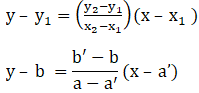Find the equations to the diagonals of the rectangle the equations of whose sides are x = a, x = a’, y = b and y = b’.

Asked by Aaryan | 1 year ago |  55

##### Solution :-

Given:

The rectangle formed by the lines x = a, x = a’, y = b and y = b’

It is clear that, the vertices of the rectangle are A (a, b), B (a’, b), C (a’, b’) and D (a, b’) .

The diagonal passing through A (a, b) and C (a’, b’) is

By using the formula,

(a’ – a)y – b(a’ – a) = (b’ – b)x – a(b’ – b)

(a’ – a) – (b’ – b)x = ba’ – ab’

Similarly, the diagonal passing through B (a’, b) and D (a, b’) is

By using the formula,(a’ – a)y – b(a – a’) = (b’ – b)x – a’ (b’ – b)

(a’ – a) + (b’ – b)x = a’b’ – ab

The equation of diagonals are y(a’ – a) – x(b’ – b) = a’b – ab’ and

y(a’ – a) + x(b’ – b) = a’b’ – ab

Answered by Sakshi | 1 year ago

### Related Questions

#### Find the angle between the line joining the points (2, 0), (0, 3) and the line x + y = 1.

Find the angle between the line joining the points (2, 0), (0, 3) and the line x + y = 1.

#### Prove that the points (2, -1), (0, 2), (2, 3) and (4, 0) are the coordinates of the vertices

Prove that the points (2, -1), (0, 2), (2, 3) and (4, 0) are the coordinates of the vertices of a parallelogram and find the angle between its diagonals.

#### Find the acute angle between the lines 2x – y + 3 = 0 and x + y + 2 = 0.

Find the acute angle between the lines 2x – y + 3 = 0 and x + y + 2 = 0.